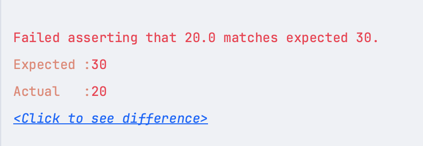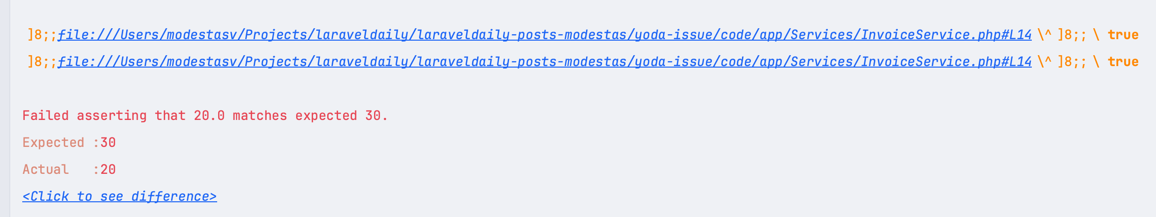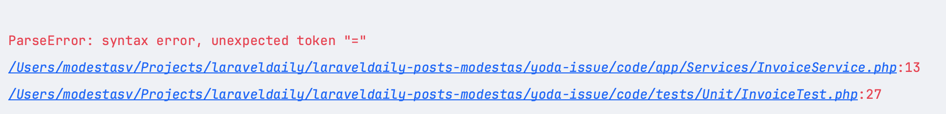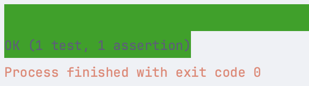# PHP: YODA-Style Comparison and Automated Tests

Have you ever seen a PHP code with an `if` statement written "backwards", like `if ("value" == \$variable)`?

``if (1 == \$variable) {    // do something}``

Why is it written this way? Why not the usual way, like `(\$variable == 1)`?

The short answer is to avoid mistyping the "=" instead of the "==" condition.

Let's take a quick example to understand this.

We will create a Service method that calculates the total of an invoice, which is the sum of all items:

``class InvoiceService{    public function getInvoiceTotal(array \$items): float    {        \$sum = 0;         foreach (\$items as \$item) {            \$itemPrice = \$item['price'] * \$item['quantity'];            // If on special sale, we need to discount it            if (\$item['specialSale'] = true) {                \$itemPrice *= \$item['specialSaleDiscount'] / 100;            }            \$sum += \$itemPrice;        }         return \$sum;    }}``

The condition here is that if the "special sale" is true, we have a different price. But have you noticed the mistake/typo in the code above?

Yup, it's this:

``if (\$item['specialSale'] = true) {``

The correct code is with `==`.

``if (\$item['specialSale'] == true) {``

But the worst part is that Laravel/PHP would not throw any errors in our incorrect code, as the "=" operator is also valid!

That's why it's very easy to leave this mistake unnoticed.

So, one way to avoid this silly mistake is to turn the code around. We can use YODA style comparison:

``if (true == \$item['specialSale']) {``

## Better Way: Automated Tests

But we don't have to write the code "backwards" and make it less readable. A better (or additional) way to check our code for bugs is to have automated tests for various cases!

So, let's write the test for both cases - with and without the "special sale":

``public function test_invoice_returns_correct_total(){    \$items = [        [            'price' => 10,            'quantity' => 2,            'specialSale' => false,            'specialSaleDiscount' => 50        ],        [            'price' => 10,            'quantity' => 2,            'specialSale' => true,            'specialSaleDiscount' => 50        ],    ];     \$this->assertEquals(30, (new InvoiceService())->getInvoiceTotal(\$items));}``

We are expecting to have 30 as our answer with the above test. This is because:

• Item 1 -> `2 * 10 = 20`
• Item 2 -> `2 * 10 = 20`, but it is on discount, so we need to discount it by 50%, so `20 * 0.5 = 10`
• Total -> `30`

Let's run the test and see what happens:It failed?! How come? Our math is correct! Let's debug our code:

``foreach (\$items as \$item) {    \$itemPrice = \$item['price'] * \$item['quantity'];    if (\$item['specialSale'] = true) {        dump(\$item['specialSale']);        \$itemPrice *= \$item['specialSaleDiscount'] / 100;    }    \$sum += \$itemPrice;}``

We added a `dump()` to see what value `\$item['specialSale']` has. Let's rerun the test:What is this? Is it `true` for both items? But our test says explicitly that the second item is on sale. Let's look at the code again:

``if (\$item['specialSale'] = true) {    \$itemPrice *= \$item['specialSaleDiscount'] / 100;}``

Did you see what happened here? We used `=` instead of `==` or even `===` and. This sets the `specialSale` to `true` instead of comparing it to `true`. How can we prevent this?

``if (true = \$item['specialSale']) {    \$itemPrice *= \$item['specialSaleDiscount'] / 100;}``

Now, if we rerun the test, we will get an error:This tells us where to fix our code. Let's fix it:

``if (true === \$item['specialSale']) {    \$itemPrice *= \$item['specialSaleDiscount'] / 100;}``

And now, if we rerun the test, it will pass:Of course, this is one of many ways to solve this issue. We could have used `===` instead of `==`, and in the worst case, we would only have a less strict comparison.

But this is just a simple example. In real life, you might have a more complex condition that is hard to debug. Using YODA style comparison can help you prevent such issues, but it's always a good practice to have the cases covered with automated tests.Very good strategy to use yoda inside tests

## Like our articles?

What else you will get:
• 52 courses (943 lessons, total 46 h 42 min)
• 75 long-form tutorials (one new every week)